# Linear algebra… with diagrams

Rediscover linear algebra by playing with circuit diagramsWires on the London Underground. CGP Grey, CC-BY 2.0

A succinct—if somewhat reductive—description of linear algebra is that it is the study of vector spaces over a field, and the associated structure-preserving maps known as linear transformations. These concepts are by now so standard that they are practically fossilised, appearing unchanged in textbooks for the best part of a century.

While modern mathematics has moved to more abstract pastures, the theorems of linear algebra are behind a surprising number of world-changing technologies: from quantum computing and quantum information, through control and systems theory, to big data and machine learning. All rely on various kinds of circuit diagrams, eg electrical circuits, quantum circuits or signal flow graphs. Circuits are geometric/topological entities, but have a vital connection to (linear) algebra, where the calculations are usually carried out.

In this article, we cut out the middle man and rediscover linear algebra itself as an algebra of circuit diagrams. The result is called graphical linear algebra and, instead of using traditional definitions, we will draw lots of pictures. Mathematicians often get nervous when given pictures, but relax: these ones are rigorous enough to replace formulas.

## Diagrams

Let’s start with a picture of a generic wire. This is our first diagram.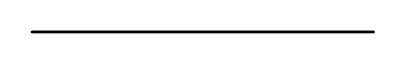(W)

We say that (W) is of type $(1,1)$ because, as a diagram, it has one dangling end on the left, and one on the right. Diagrams can be stacked on top of one another; for example, the following is obtained by stacking (W) on top of itself, obtaining a $(2,2)$ diagram.Wires can be be jumbled up, with the following $(2,2)$ diagram called a twist.(T)

In addition to stacking, diagrams can also be connected in series if the numbers of wires agree on the connection boundary. The following results from connecting two twists.It is useful to imagine that the wires are stretchy, like rubber bands.
For example, we consider the following three $(4,4)$ diagrams to be equal.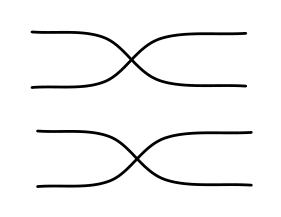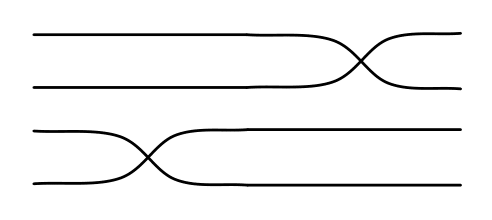We will need additional equations between diagrams. A nice value-added feature of the notation is that equations often convey topological intuitions: eg we require that a twist followed by a twist can be ‘untangled’ in the following sense.In some settings, eg knot theory, the above equation would not be imposed because knots—unsurprisingly—rely on the ability of wires to tangle. (See Dan Ghica’s entertaining Inventing a knot theory for eight year olds).

The final thing to say about twists is that we can ‘pull’ diagrams across them, preserving equality. The following, known as the Yang–Baxter equation, is an instance of this: we pull the top left twist across the third wire, which, for sake of legibility, is coloured red.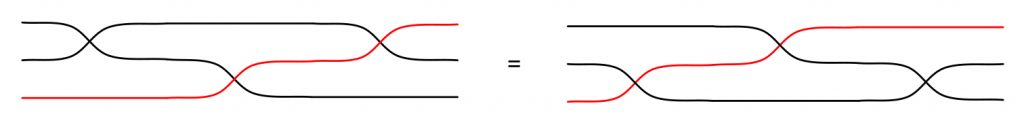(YB)

Given these insights, it is not too difficult to prove that the diagrams we’ve seen so far are in bijective correspondence with permutations. For example, those in (YB) correspond to the permutation $\rho$ on the three element set $\{0,1,2\}$ where $\rho(x)=2-x$.

An optional note for category theory aficionados: our diagrams are the arrows of a strict symmetric monoidal category with objects the natural numbers. The arrows from $m$ to $n$ are $(m,n)$ diagrams. All diagrams in this article are arrows of such categories, which are called PROPs. Thus ‘pulling’ diagrams across twists, as in (YB), is none other than the naturality of the braiding structure and (S) ensures that the braiding is a symmetry.

## Copying

Let’s imagine that wires carry data from left to right. Whatever it is, assume that we can copy it. To copy, we get a special $(1,2)$ contraption, illustrated below, which takes data from the wire on the left and copies it to the two wires on the right.A defining feature of copies is that one should not be able to distinguish between them; copying, then swapping, is the same as just copying. We thus add the following:If I copy twice, I end up with three copies. There are two ways to do this, but they are indistinguishable. The following equation ensures this: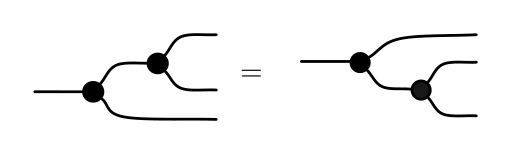The ability to copy often comes with the ability to throw away, otherwise photocopying rooms would quickly fill up with scrap. Throwing away is done by the $(1,0)$ diagram below, which takes data and returns nothing.Finally, if I copy and throw away one copy, I haven’t achieved very much in the grand scheme of things. This is the intuition behind the following equation.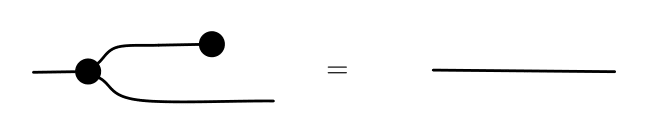The above structure is otherwise known as a (cocommutative) comonoid. Copying is awkward to denote with standard formula syntax. For example, $f(x,y)$ is a standard way of denoting an operation that takes two arguments and returns one result. But how can we denote an operation that takes one argument and returns two results? The kinds of diagrams that we have seen so far are a solution.

Next, we imagine that data on the wires can be added. For the sake of concreteness, we may as well assume that wires carry integers. Then a $(2,1)$ addition operation takes two arguments and has a single result.Adding comes with an identity element, and we introduce a $(0,1)$ gadget for this.The intuition is that the diagram above outputs 0 on its result wire. Given that addition is commutative, associative and has an identity element, the following equations ought to be uncontroversial.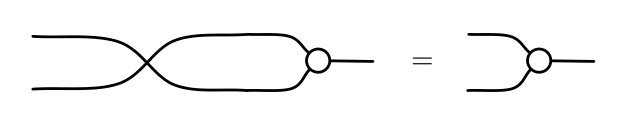The above structure is otherwise known as a (commutative) monoid. An intriguing thing about the diagrammatic notation is that, ignoring the black/white colouring, the equations involved for monoids are mirror images of those for comonoids.

The fun really starts when diagrams combine adding and copying. From now on, we will draw diagrams that feature all of the following:copy throw away add output 0

subject to both the comonoid and monoid equations we considered before. But now the two structures can connect to each other, leading to some new and interesting situations.

First, when we copy zero, we get two zeros. Similarly, when we discard the result of addition, it’s the same as discarding the arguments. This leads to the following: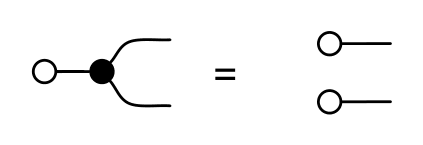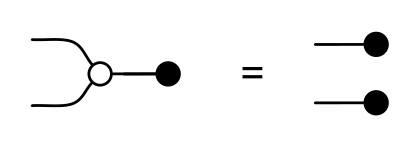One of the most interesting equations concerns copying the result of an addition: it is the same as if we copied the arguments and performed two additions, separately.The last equation is about discarding zero. The effect is… nothing, the empty diagram.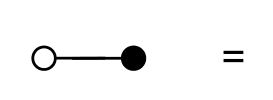This is the point in the story where linear algebra starts bubbling up to the surface. Diagrams—with all of the equations we have considered so far—are now in bijective correspondence with matrices of natural numbers. Moreover, composing diagrams in series corresponds to matrix multiplication, and stacking two diagrams to a direct sum.

At first sight, this may seem a little bit magical: we drew diagrams by stacking and connecting basic components; we never even defined the natural numbers! Moreover, multiplying matrices involves ordinary addition and multiplication of integers. So where is all of this structure hiding in the diagrams?

The best way to get the idea is via examples. Following the correspondence, the $(1,1)$ diagrams below ought to be $1\times 1$ matrices. In fact, they correspond to $(0)$, $(1)$, $(2)$ and $(5)$. Notice the pattern? To get the number, count the paths from the left to the right.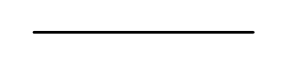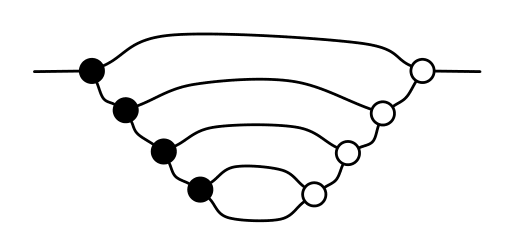Can you figure out how to multiply and add numbers, as diagrams? If yes, you’ll enjoy proving that multiplication distributes over addition. (If you get stuck, it’s all explained on the graphical linear algebra website). This is the first example of a common phenomenon in graphical linear algebra: basic algebraic operations—often considered primitive—are actually instances of the algebra of diagrams.
One final example: the $2\times 3$ matrix
\begin{equation*}
\begin{pmatrix}
1 & 0 & 2 \\
0 & 1 & 1
\end{pmatrix}
\end{equation*}
corresponds to the $(3,2)$ diagram below. To get the $(i,j)$th entry, count the number of paths from the $j$th input to the $i$th output.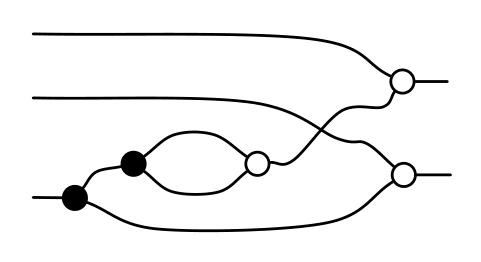This algebraic structure is known as a (bicommutative) bimonoid, or bialgebra. Bimonoids are common all over mathematics, eg in algebra, combinatorics, topology and models of computation. Once familiar with this pattern of interaction between a monoid and comonoid, you will see it everywhere.

The most exciting part of the story comes when we confuse the ‘direction of flow’ in diagrams. This means, roughly speaking, that copying and adding can now go both from left to right, and from right to left. Our diagrams will now feature all ofwith all of the equations considered so far.

The way to make sense of this is to stop considering the left side of the diagrams as ‘inputs’ and the right side as ‘outputs’. Technically, it means not thinking of addition and copying as functions, but rather as relations, which, unlike functions, can always be reflected. For example, addition—as a relation—is the set of pairs
$\left(\begin{pmatrix} x\\ y\end{pmatrix} ,\, x+y\right),$
while ‘backwards’ addition is the relation
$\left(x+y,\, \begin{pmatrix}x\\ y\end{pmatrix}\right).$
As before, this leads to several new situations to consider. Intriguingly, it turns out that the same equations describe the interaction of copying and adding with their mirror images.The above are known as Frobenius equations, and are the other common way that monoids and comonoids interact. Just as for bimonoids, this pattern of interaction can be found in many different places, all over mathematics and its related fields.

Enumerating all the equations would be an overkill for this article, so let’s go straight to the punchline. Previously, our diagrams were a bijection with matrices of natural numbers, even if it took some mental yoga to see the correspondence. This time, the magic ramps up a few notches: diagrams are now in bijective correspondence with linear relations over the field $\mathbb{Q}$ of the rational numbers, AKA fractions. But let’s go through this step by step.

First, where do fractions come from? Before, when everything flowed from left to right, there was a bijection between $(1,1)$ diagrams and natural numbers. But with mirror images of copying and adding around, direction of flow is confused, bringing additional expressivity. For example, the following $(1,1)$ diagram is the diagrammatic way of writing $\frac{2}{3}$: it connects a $2$, going from left to right, with a $3$ going from right to left.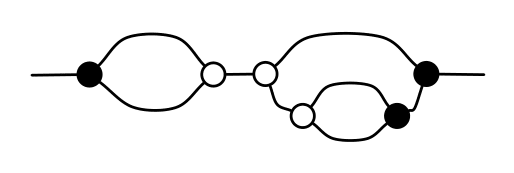($\frac23$)

Just as the multiplication and addition of natural numbers can be derived from the algebra of diagrams, so can the algebra of fractions that everyone learns in primary school. As it turns out, not all diagrams of type $(1,1)$ are fractions, which leads us to an interesting feature of graphical linear algebra. But first, a little detour.

A curious phenomenon of human languages is that some words are notoriously difficult to translate. Some have become quite well-known, almost cliché: Schadenfreude in German, furbo in Italian or hygge in Danish. This is a side effect of the subtle differences in expressivity between languages: a concept natural in one is sometimes clumsy to express in another. Differences in expressivity also show up in formal languages.

With this in mind—and please don’t freak out—the algebra of diagrams has nothing to stop you from dividing by zero. This is because reflecting a $(1,1)$ diagram means taking the reciprocal: eg $3$ is the diagram with three paths from left to right, and $\frac{1}{3}$ is the diagram with three paths from right to left. Keeping this in mind, the following would seem to be the diagram for $\frac{1}{0}$.(∞)

It’s natural to be a bit nervous, considering the anti division-by-zero propaganda bombarding us from a young age: as a result, most of us suffer from an acute dividebyzerophobia. But nothing explodes, and it can actually be useful to work in this extended algebra of fractions, featuring division by zero. To tie up the story, let’s complete the taxonomy of $(1,1)$ diagrams: there is a diagram for each ordinary fraction, the diagram (∞) above and just two additional diagrams, illustrated below, which can be understood as two different ways of translating $\frac{0}{0}$ to the language of diagrams. (More dividing by zero on the graphical linear algebra website).($\bot$)($\top$)

In general, $(m,n)$ diagrams are in bijective correspondence with linear relations: those subsets of $\mathbb{Q}^m\times\mathbb{Q}^n$ that are closed under pointwise addition and $\mathbb{Q}$ multiplication, ie that are $\mathbb{Q}$ vector spaces. For example, the linear relation of (∞) is $\{\,(0,q) \,:\, q\in\mathbb{Q}\,\}$, and those of ($\bot$ and $\top$) are, respectively, $\{\,(0,0)\,\}$ and $\{\,(p,q) \,:\, p,q\in\mathbb{Q}\,\}$. The linear relation for ($\frac23$) is $\{\,(3p,2p)\,:\, p\in\mathbb{Q}\,\}$. Other examples of such beasts include kernels and images of matrices with $\mathbb{Q}$ entries; all of which are thus expressible with the language of diagrams. Now, since all of the diagrammatic equations involve only adding and copying operations, we conclude with an insight that’s not so apparent given the usual way of presenting linear algebra:

Linear algebra is what happens when adding meets copying.

[Banner image: Vera de Kok, CC BY 2.0]Paweł is a theoretical computer scientist at the University of Southampton, focusing on compositional modelling of systems, developing the underlying maths (usually category theory), and applying it to real-life problems such as verification. Since 2015, he has been working on the Graphical Linear Algebra blog, rediscovering linear algebra with string diagrams.
https://graphicallinearalgebra.net/    + More articles by Paweł